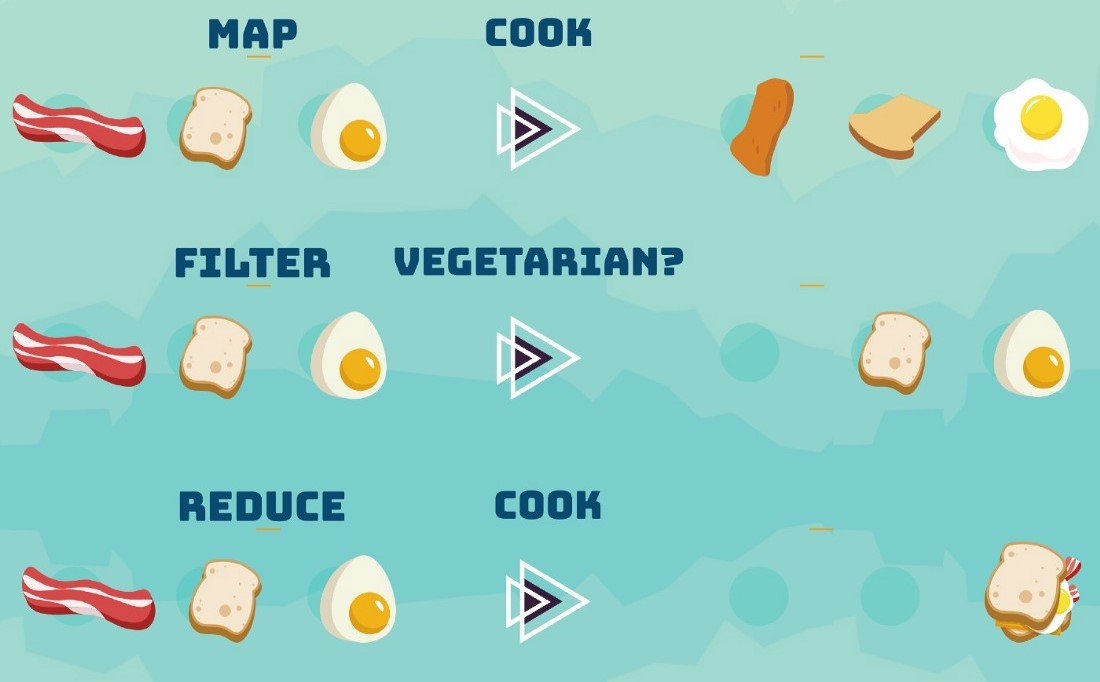# Learning Python with Advent of Code Walkthroughs

Dazbo's Advent of Code solutions, written in Python

# The Python Journey - Map, Filter, and Reduce## map

The `map()` function applies a function against every member in a sequence. The function takes two arguments:

1. The function we need to apply to each member of the iterable, without the parentheses.
2. The iterable itself.

### Example: Converting from Str to Int

Here we apply the `int()` function to each member of a list. The original list contains str values.

``````def print_seq(seq):
for item in seq:
print(f"{item}, type: {type(item)}")

sequence = ['1', '2', '3', '4', '5'] # list of str values
print("Items in sequence...")
print_seq(sequence)

new_seq = map(int, sequence) # convert each str to an int
print("Items in new_seq...")
print_seq(new_seq)
``````

Output:

``````Items in sequence...
1, type: <class 'str'>
2, type: <class 'str'>
3, type: <class 'str'>
4, type: <class 'str'>
5, type: <class 'str'>
Items in new_seq...
1, type: <class 'int'>
2, type: <class 'int'>
3, type: <class 'int'>
4, type: <class 'int'>
5, type: <class 'int'>
``````

### Example: Getting Word Lengths

Here we split a phrase into its constituent words. Then we apply the `len()` function to each word, to determine the length of each word.

``````PHRASE = "The quick brown fox jumped over the lazy dog"
words = PHRASE.split()

word_lengths = map(len, words)
for word, word_len in zip(words, word_lengths):
print(f"Word: {word}, length: {word_len}")
``````

Output:

``````Word: The, length: 3
Word: quick, length: 5
Word: brown, length: 5
Word: fox, length: 3
Word: jumped, length: 6
Word: over, length: 4
Word: the, length: 3
Word: lazy, length: 4
Word: dog, length: 3
``````

## filter

The `filter()` function applies a function to each member of an iterable. The function it applies must always return a `boolean`. If the function returns `True`, then this member of the iterable is included in the iterable returned by the `filter()` function itself. If the function returns `False`, then this member is not included.

TL;DR: We can use `filter` to apply filtering criteria to a collection, and only return those items that meet the criteria.

As with `map()`, the `filter()` function takes two parameters:

1. The function that is used to filter our collection, without the parentheses. It must return a boolean.
2. The iterable itself.

### Example: Filtering Based on Word Length

If we continue our example from above, we could write a filter to only return words that have 5 characters or more:

``````long_words = list(filter(lambda x: len(x) >= 5, words))
print(long_words)
``````

Output:

``````['quick', 'brown', 'jumped']
``````

Note that we’ve used a lambda function as the first parameter to `filter()`.

## reduce

The `reduce()` function applies a function successively to each element in an iterable. It starts by applying the function to elements 1 and 2, resulting in a so-called aggregator value. It then applies the function to the aggregator value and element 3, resulting in a new aggregator value. Then it applies the function to the new aggregator value and element 4, and so on.

Ultimately, the `reduce()` function reduces the iterable down to one final value.

The `reduce()` function is often called `fold` in other languages.

As with the `map()` and `filter()` functions, `reduce()` takes two parameters:

1. The function that is applied sequentially, without the parentheses. This function must itself accept two parameters.
2. The iterable itself.

### Example: Using Reduce() to Implement a Factorial Function

``````from functools import reduce

def mul(a, b):
prod = a * b
print(f"{a} * {b} = {prod}") # so we can see the interim values
return prod

fac = reduce(mul, range(1, 11))
print(f"Result = {fac}")
``````

Output:

``````1 * 2 = 2
2 * 3 = 6
6 * 4 = 24
24 * 5 = 120
120 * 6 = 720
720 * 7 = 5040
5040 * 8 = 40320
40320 * 9 = 362880
362880 * 10 = 3628800
Result = 3628800
``````

Note that to use `reduce()`, it has to be imported from `functools`.